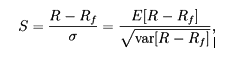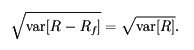# Sharp Ratio

The Sharpe ratio or Sharpe index or Sharpe measure or reward-to-variability ratio is a measure of the excess return (or Risk Premium) per unit of risk in an investment asset or a trading strategy, named after William Forsyth Sharpe. Since its revision by the original author in 1994, it is defined as:where R is the asset return, Rf is the return on a benchmark asset, such as the risk free rate of return, E[R − Rf] is the expected value of the excess of the asset return over the benchmark return, and σ is the standard deviation of the asset.

Note, if Rf is a constant risk free return throughout the period,The Sharpe ratio is used to characterize how well the return of an asset compensates the investor for the risk taken, the higher the Sharpe ratio number the better. When comparing two assets each with the expected return E[R] against the same benchmark with return Rf, the asset with the higher Sharpe ratio gives more return for the same risk. Investors are often advised to pick investments with high Sharpe ratios. However like any mathematical model it relies on the data being correct. Pyramid schemes with a long duration of operation would typically provide a high Sharpe ratio when derived from reported returns but the inputs are false.